# Real Estate Principles Study Set 1

## Quiz 15 : Mortgage Calculations and DecisionsStudy FlashcardsLooking for Insurance Homework Help?

## Quiz 15 :Mortgage Calculations and Decisions

Question TypeSuppose a one-year ARM loan has a margin of 2.75 percent, a teaser rate for the first year of 4.00 percent, and a cap of 1.00 percent. If the index rate is 3.00 percent at the end of the first year, what will be the interest rate on the loan in year 2 If there is more than one possible answer, what does the outcome depend on
Free
Essay

We are asked to consider the following variables: Suppose a one-year ARM loan has a margin of 2.75 percent, a teaser rate for the first year of 4.00 percent, and a cap of 1.00 percent.
If the index rate is 3.00 percent at the end of the first year, what will be the interest rate on the loan in year 2 If there is more than one possible answer, what does the outcome depend on
If the periodic cap applies to the beginning or opening rate, in year two the interest rate will be limited to 5.00 percent. If the cap applies only to margin plus index, in year two the interest rate would be 5.75 percent.

Tags
Choose question tagA homeowner is attempting to decide between a 15-year mortgage loan at 5.5 percent and a 30-year loan at 5.9 percent. What would you advise What would you advise if the borrower also has a large amount of credit card debt outstanding at a rate of 15 percent
Free
Essay

We are asked to consider the following variables: A homeowner is attempting to decide between a 15-year mortgage loan at 5.5 percent and a 30-year loan at 5.90 percent. What would you advise What would you advise if the borrower also has a large amount of credit card debt outstanding at a rate of 15 percent
If the borrower is not carrying a large debt at a rate significantly higher than the rates on the loan, then the variance in the mortgage rates can be considered to be a maturity premium difference. Bearing this information in mind, the borrower can see the loans as roughly the same on a purely financial basis.
If the borrower is carrying a large consumer debt at a rate significantly higher, then a longer term loan may be preferable. So, if the debt rates of the consumer credit are significantly higher than the mortgage interest rate, it would be better to choose the longer-term mortgage loan.

Tags
Choose question tagAssume the following for a one-year adjustable rate mortgage loan that is tied to the one-year Treasury rate: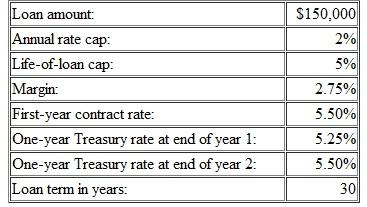Given these assumptions, calculate the following: a. Initial monthly payment. b. Loan balance end of year 1. c. Year 2 contract rate. d. Year 2 monthly payment. e. Loan balance end of year 2. f. Year 3 contract rate. g. Year 3 payment
Free
Essay

We are asked to calculate the following variables: Assume the following for a one-year rate adjustable rate mortgage loan that is tied to the one-year Treasury rate:
Loan amount: $150,000 Annual rate cap: 2% Life-of-loan cap: 5% Margin: 2.75% First-year contract rate: 5.50% One-year Treasury rate at end of year 1: 5.25% One-year Treasury rate at end of year 2: 5.50% Loan term in years: 30 Given these assumptions, calculate the following for a. through g.: (a) Using the information in the introduction, we need to calculate an initial monthly payment. Using your financial calculator, please enter the values as shown in Table 1 to solve for the initial monthly payment. Table 1: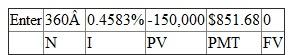Solve for PMT =$851.68
Your calculator should display the first year payment, based on an interest rate of 5.5 percent, to equal $851.68. (b) Using the information in the introduction, we need to calculate the loan balance at the end of year 1. Using your financial calculator, please enter the values as shown in Table 2 to solve for the loan balance at the end of year 1. Table 2: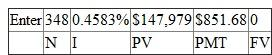Solve for PV =$147,979
Your calculator should display the loan balance at the end of year one equals $147,979. (c) Using the information in the introduction, we need to calculate the contract rate for year two. We will use the annual cap that applies to the teaser rate in our calculations. Our solution equals a 7.50 percent interest rate in year. (d) Using the information in the introduction, we need to calculate the monthly payment for Year 2. Using your financial calculator, please enter the values as shown in Table 3 to solve for monthly payment for Year 2. Table 3: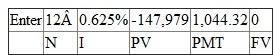Solve for PMT =$1,044.32
Your calculator should display the monthly payment for Year 2 as equal to $1,044.32 considering the following variables: • remaining term of 29 years • interest rate of 7.5 percent • balance of$147,979.41
(e) Using the information in the introduction, we need to calculate the Loan balance end of year 2. Using your financial calculator, please enter the values as shown in Table 4 to solve for the loan balance at the end of Year 2.
Table 4: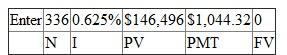Solve for PV = $146,496 Your calculator should display the loan balance at the end of year two as equal to$146,496.
(f) Using the information in the introduction, we need to calculate the contract rate for Year 3. We know that we need to use the interest rate is index plus margin, or 8.25 percent to find the year three contract rate.
(g) Using the information in the introduction, we need to calculate the Year 3 payment. Based on a balance of $146,496, the year three payment is$1,119, considering the remaining term of 28 years and an interest rate of 8.25 percent.

Tags
Choose question tagGive some examples of up-front financing costs associated with residential mortgages. What rule can one apply to determine if a settlement (closing) cost should be included in the calculation of the effective borrowing cost
Essay
Tags
Choose question tagOn an adjustable rate mortgage, do borrowers always prefer smaller (tighter) rate caps that limit the amount the contract interest rate can increase in any given year or over the life of the loan Explain why or why not.
Essay
Tags
Choose question tagOn the following loan, what is the best estimate of the effective borrowing cost if the loan is prepaid in six years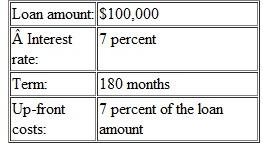Multiple Choice
Tags
Choose question tagFor a loan of $100,000, at 7percent interest for 30 years, find the balance at the end of 4 years and 15 years. Essay Answer: Tags Choose question tagAdjustable rate mortgages (ARMs) commonly have all the following except: Multiple Choice Answer: Tags Choose question tagAssume the following: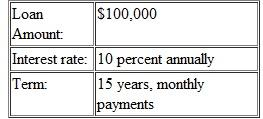a. What is the monthly payment b. What will be the loan balance at the end of nine years c. What is the effective borrowing cost on the loan if the lender charges 3 points at origination and the loan goes to maturity d. What is the effective borrowing cost on the loan if the lender charges 3 points at origination and the loan is prepaid at the end of year 9 Essay Answer: Tags Choose question tagA mortgage banker is originating a level-payment mortgage with the following terms: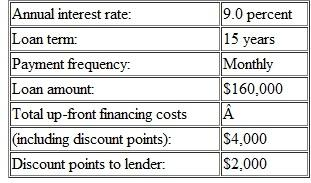a. Calculate the annual percentage rate (APR) for Truth-in- Lending purposes. b. Calculate the lender's yield with no prepayment. c. Calculate the lender's yield with prepayment in five years. d. Calculate the effective borrowing cost with prepayment in five years. Essay Answer: Tags Choose question tagThe annual percentage rate (APR) was created by: Multiple Choice Answer: Tags Choose question tagConsider a$75,000 mortgage loan with an annual interest rate of 8 percent. The loan term is seven years, but monthly payments will be based on a 30-year amortization schedule. What is the monthly payment What will be the balloon payment at the end of the loan term
Essay
Tags
Choose question tagLender's yield differs from effective borrowing cost (EBC) because:
Multiple Choice
Tags
Choose question tagA characteristic of a partially amortized loan is:
Multiple Choice
Tags
Choose question tagThe dominant loan type originated by most financial institutions is the:
Multiple Choice
Tags
Choose question tagWhich of the following statements is true about 15- and 30-year fixed-payment mortgages
Multiple ChoiceCalculate the original loan size of a fixed-payment mortgage if the monthly payment is $1,146.78, the annual interest rate is 8.0 percent, and the original loan term is 15 years. Essay Answer: Tags Choose question tagIf a mortgage is to mature (i.e., become due) at a certain future time without any reduction in principal, this is called: Multiple Choice Answer: Tags Choose question tagThe most typical adjustment interval on an adjustable rate mortgage (ARM) once the interest rate begins to change is: Multiple Choice Answer: Tags Choose question tagOn a level-payment loan with 12 years (144 payments) remaining, at an interest rate of 9 percent, and with a payment of$1,000, the balance is: